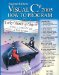# B.5. Converting from Decimal to Binary, Octal or Hexadecimal

The conversions in Section B.4 follow naturally from the positional notation conventions. Converting from decimal to binary, octal, or hexadecimal also follows these conventions.

Suppose we wish to convert decimal 57 to binary. We begin by writing the positional values of the columns right to left until we reach a column whose positional value is greater than the decimal number. We do not need that column, so we discard it. Thus, we first write:

 Positional values: 64 32 16 8 4 2 1

Then we discard the column with positional value 64, leaving:

 Positional values: 32 16 8 4 2 1

Next we work from the leftmost column to the right. We divide 32 into 57 and observe that there is one 32 in 57 with a remainder of 25, so we write 1 in the 32 column. We divide 16 into 25 and observe that there is one 16 in 25 with a remainder of 9 and write 1 in the 16 column. We divide 8 into 9 and observe that there is one 8 in 9 with a remainder of 1. The next two columns each produce quotients of 0 when their positional values are divided into 1, so we write 0s in the 4 and 2 columns. Finally, 1 into 1 is 1, so we write 1 in the 1 column. This yields:

 Positional values: 32 16 8 4 2 1 Symbol values: 1 1 1 0 0 1

and thus decimal 57 is equivalent to binary 111001.

To convert decimal 103 to octal, we begin by writing the positional values of the columns until we reach a column whose positional value is greater than the decimal number. We do not need that column, so we discard it. Thus, we first write:

 Positional values: 512 64 8 1

Then we discard the column with positional value 512, yielding:

 Positional values: 64 8 1

Next we work from the leftmost column to the right. We divide 64 into 103 and observe that there is one 64 in 103 with a remainder of 39, so we write 1 in the 64 column. We divide 8 into 39 and observe that there are four 8s in 39 with a remainder of 7 and write 4 in the 8 column. Finally, we divide 1 into 7 and observe that there are seven 1s in 7 with no remainder, so we write 7 in the 1 column. This yields:

 Positional values: 64 8 1 Symbol values: 1 4 7

and thus decimal 103 is equivalent to octal 147.

To convert decimal 375 to hexadecimal, we begin by writing the positional values of the columns until we reach a column whose positional value is greater than the decimal number. We do not need that column, so we discard it. Thus, we first write:

 Positional values: 4096 256 16 1

Then we discard the column with positional value 4096, yielding:

 Positional values: 256 16 1

Next we work from the leftmost column to the right. We divide 256 into 375 and observe that there is one 256 in 375 with a remainder of 119, so we write 1 in the 256 column. We divide 16 into 119 and observe that there are seven 16s in 119 with a remainder of 7 and write 7 in the 16 column. Finally, we divide 1 into 7 and observe that there are seven 1s in 7 with no remainder, so we write 7 in the 1 column. This yields:

 Positional values: 256 16 1 Symbol values: 1 7 7

and thus decimal 375 is equivalent to hexadecimal 177.Visual C# 2005 How to Program (2nd Edition)
ISBN: 0131525239
EAN: 2147483647
Year: 2004
Pages: 600

Similar book on Amazon

Flylib.com © 2008-2020.
If you may any questions please contact us: flylib@qtcs.net